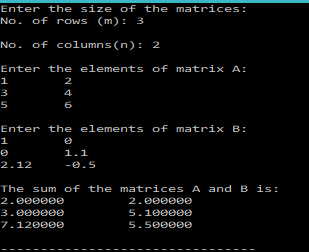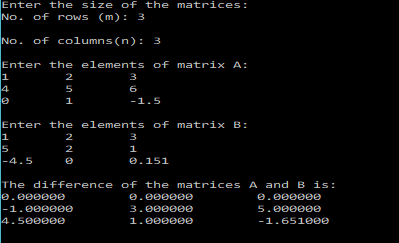# C Program for Adding or Subtracting Matrices

In the last post I discussed the concept of arrays in C.
One can define matrices in C using 2-D arrays.

In this post I will assume, that you are familiar with the concepts of arrays.

We know, that if two matrices, A and B are of same size(order), that is, they have the same no. of rows and columns, then they can be added or subtracted.

Mathematically,

Let$A$ and$B$ be two matrices of order$m\times n$. Then their sum/difference is given as:$(A \pm B)_{ij}= a_{ij} \pm b_{ij}$

Using the above information we can write a simple C program that asks the user to enter the order of the matrices, and then prompts the user to enter the elements of the matrices row-wise, and finally print the sum/difference.

The program for Matrix Addition is as shown below:

### CODE:

/**************************************************
**************************************************/
#include<stdio.h>
main(){
int m,n,i,j;
printf("Enter the size of the matrices:\nNo. of rows (m): ");
scanf("%d",&m);
printf("\nNo. of columns(n): ");
scanf("%d",&n);
double a[m][n];
double b[m][n];
double sum[m][n];
printf("\nEnter the elements of matrix A:\n");
for(i=0;i<m;i++){
for(j=0;j<n;j++){
scanf("%lf",&a[i][j]);
}
}
printf("\nEnter the elements of matrix B:\n");
for(i=0;i<m;i++){
for(j=0;j<n;j++){
scanf("%lf",&b[i][j]);
}
}
for(i=0;i<m;i++){
for(j=0;j<n;j++){
sum[i][j]=a[i][j]+b[i][j];
}
}
printf("\nThe sum of the matrices A and B is:\n");
for(i=0;i<m;i++){
for(j=0;j<n;j++){
printf("%lf \t",sum[i][j]);
}
printf("\n");
}
}


In the above code, we ask the user to enter the size of the matrices and store the information in m and n. Then declare three 2-D arrays(matrices) of the given size, and ask the user to enter the matrix entries.
Then we sum the matrices, and print the answer.

### OUTPUT:

A sample output for the above code is:Similarly, one can write program to calculate the Matrix Difference, as shown below:

### CODE:

/**************************************************
***************MATRIX SUBTRACTION******************
**************************************************/
#include<stdio.h>
main(){
int m,n,i,j;
printf("Enter the size of the matrices:\nNo. of rows (m): ");
scanf("%d",&m);
printf("\nNo. of columns(n): ");
scanf("%d",&n);
double a[m][n];
double b[m][n];
double diff[m][n];
printf("\nEnter the elements of matrix A:\n");
for(i=0;i<m;i++){
for(j=0;j<n;j++){
scanf("%lf",&a[i][j]);
}
}
printf("\nEnter the elements of matrix B:\n");
for(i=0;i<m;i++){
for(j=0;j<n;j++){
scanf("%lf",&b[i][j]);
}
}
for(i=0;i<m;i++){
for(j=0;j<n;j++){
diff[i][j]=a[i][j]-b[i][j];
}
}
printf("\nThe difference of the matrices A and B is:\n");
for(i=0;i<m;i++){
for(j=0;j<n;j++){
printf("%lf \t",diff[i][j]);
}
printf("\n");
}
}


### OUTPUT:

A sample output for the above code is:Now, to make your code neater and more reusable, you would want to wrap the code to calculate the sum and difference in a separate function.
Also, one could wrap the code to read and print matrices in separate functions as well.

Now ideally, I would like a function that takes in the two matrices A and B as the parameters and returns the sum/difference matrix.
Unfortunately, C doesn’t let you return arrays(matrices) in my limited knowledge.

So one has two alternatives, to accomplish our aim.
1. We create a matrix called sum in our main program and then pass it as a parameter to the adding function, which would then populate this matrix with the sum. Note, that in C when you pass an array as a parameter, you are not passing it by value like it happens with variables, but rather you are passing a reference to the array itself. So when you pass the matrix that stores the sum, the original matrix will be modified. All of this can work out for us without much hassle.
2. Another, option would be to use pointers. We would use malloc to create our sum/diff matrix in the function to allocate sufficient space. And then return the pointer to this array. This would be a dynamic allocation.

In this post I will be going with the first method, due to it’s simplicity, and no use of pointers.

The following codes illustrate the above procedure to add two matrices.

### CODE:

/**************************************************
**************************************************/
#include<stdio.h>
/*******
Function that calculates the sum of two matrices:
There are two options to do this in C.
1. Pass a matrix (sum) as the parameter, and calculate and store the sum in it.
2. Use malloc and make the function of pointer type and return the pointer.
This program uses the first option.
********/
void matSum(int m, int n, double a[m][n], double b[m][n], double sum[m][n] ){
int i,j;
for(i=0;i<m;i++){
for(j=0;j<n;j++){
sum[i][j]=a[i][j]+b[i][j];
}
}
}
/*******
Function that reads the elements of a matrix row-wise
Parameters: rows(m),columns(n),matrix[m][n]
*******/
void readMatrix(int m, int n, double matrix[m][n]){
int i,j;
for(i=0;i<m;i++){
for(j=0;j<n;j++){
scanf("%lf",&matrix[i][j]);
}
}
}
/*******
Function that prints the elements of a matrix row-wise
Parameters: rows(m),columns(n),matrix[m][n]
*******/
void printMatrix(int m, int n, double matrix[m][n]){
int i,j;
for(i=0;i<m;i++){
for(j=0;j<n;j++){
printf("%lf\t",matrix[i][j]);
}
printf("\n");
}
}
main(){
int m,n,i,j;
printf("Enter the size of the matrices:\nNo. of rows (m): ");
scanf("%d",&m);
printf("\nNo. of columns(n): ");
scanf("%d",&n);
double a[m][n];
double b[m][n];
double sum[m][n];
printf("\nEnter the elements of matrix A:\n");
printf("\nEnter the elements of matrix B:\n");
matSum(m,n,a,b,sum);
printf("\nThe sum of the matrices A+B is:\n");
printMatrix(m,n,sum);

}


The above code contains, separate functions that perform different tasks, like addition, printing and reading of the matrices. This makes the code more readable and re-usable.

Similarly, one could write the code to subtract two matrices, by making a few changes to the above code.

### CODE:

/**************************************************
****************MATRIX SUBTRACTION*****************
**************************************************/
#include<stdio.h>
/*******
Function that calculates the difference of two matrices:
There are two options to do this in C.
1. Pass a matrix (diff) as the parameter, and calculate and store the difference in it.
2. Use malloc and make the function of pointer type and return the pointer.
This program uses the first option.
********/
void matDiff(int m, int n, double a[m][n], double b[m][n], double diff[m][n] ){
int i,j;
for(i=0;i<m;i++){
for(j=0;j<n;j++){
diff[i][j]=a[i][j]-b[i][j];
}
}
}
/*******
Function that reads the elements of a matrix row-wise
Parameters: rows(m),columns(n),matrix[m][n]
*******/
void readMatrix(int m, int n, double matrix[m][n]){
int i,j;
for(i=0;i<m;i++){
for(j=0;j<n;j++){
scanf("%lf",&matrix[i][j]);
}
}
}
/*******
Function that prints the elements of a matrix row-wise
Parameters: rows(m),columns(n),matrix[m][n]
*******/
void printMatrix(int m, int n, double matrix[m][n]){
int i,j;
for(i=0;i<m;i++){
for(j=0;j<n;j++){
printf("%lf\t",matrix[i][j]);
}
printf("\n");
}
}
main(){
int m,n,i,j;
printf("Enter the size of the matrices:\nNo. of rows (m): ");
scanf("%d",&m);
printf("\nNo. of columns(n): ");
scanf("%d",&n);
double a[m][n];
double b[m][n];
double diff[m][n];
printf("\nEnter the elements of matrix A:\n");